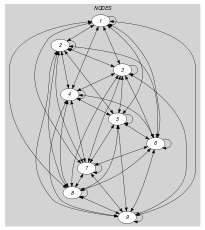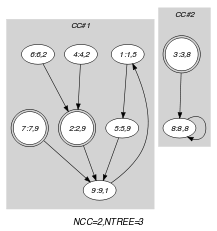## 5.239. map

Origin

Inspired by [SedgewickFlajolet96]

Constraint

$\mathrm{𝚖𝚊𝚙}\left(\mathrm{𝙽𝙱𝙲𝚈𝙲𝙻𝙴},\mathrm{𝙽𝙱𝚃𝚁𝙴𝙴},\mathrm{𝙽𝙾𝙳𝙴𝚂}\right)$

Arguments
 $\mathrm{𝙽𝙱𝙲𝚈𝙲𝙻𝙴}$ $\mathrm{𝚍𝚟𝚊𝚛}$ $\mathrm{𝙽𝙱𝚃𝚁𝙴𝙴}$ $\mathrm{𝚍𝚟𝚊𝚛}$ $\mathrm{𝙽𝙾𝙳𝙴𝚂}$ $\mathrm{𝚌𝚘𝚕𝚕𝚎𝚌𝚝𝚒𝚘𝚗}\left(\mathrm{𝚒𝚗𝚍𝚎𝚡}-\mathrm{𝚒𝚗𝚝},\mathrm{𝚜𝚞𝚌𝚌}-\mathrm{𝚍𝚟𝚊𝚛}\right)$
Restrictions
 $\mathrm{𝙽𝙱𝙲𝚈𝙲𝙻𝙴}\ge 0$ $\mathrm{𝙽𝙱𝚃𝚁𝙴𝙴}\ge 0$ $\mathrm{𝚛𝚎𝚚𝚞𝚒𝚛𝚎𝚍}$$\left(\mathrm{𝙽𝙾𝙳𝙴𝚂},\left[\mathrm{𝚒𝚗𝚍𝚎𝚡},\mathrm{𝚜𝚞𝚌𝚌}\right]\right)$ $\mathrm{𝙽𝙾𝙳𝙴𝚂}.\mathrm{𝚒𝚗𝚍𝚎𝚡}\ge 1$ $\mathrm{𝙽𝙾𝙳𝙴𝚂}.\mathrm{𝚒𝚗𝚍𝚎𝚡}\le |\mathrm{𝙽𝙾𝙳𝙴𝚂}|$ $\mathrm{𝚍𝚒𝚜𝚝𝚒𝚗𝚌𝚝}$$\left(\mathrm{𝙽𝙾𝙳𝙴𝚂},\mathrm{𝚒𝚗𝚍𝚎𝚡}\right)$ $\mathrm{𝙽𝙾𝙳𝙴𝚂}.\mathrm{𝚜𝚞𝚌𝚌}\ge 1$ $\mathrm{𝙽𝙾𝙳𝙴𝚂}.\mathrm{𝚜𝚞𝚌𝚌}\le |\mathrm{𝙽𝙾𝙳𝙴𝚂}|$
Purpose

Number of trees and number of cycles of a map. We take the description of a map from  [SedgewickFlajolet96]:

Every map decomposes into a set of connected components, also called connected maps. Each component consists of the set of all points that wind up on the same cycle, with each point on the cycle attached to a tree of all points that enter the cycle at that point.

Example
$\left(\begin{array}{c}2,3,〈\begin{array}{cc}\mathrm{𝚒𝚗𝚍𝚎𝚡}-1\hfill & \mathrm{𝚜𝚞𝚌𝚌}-5,\hfill \\ \mathrm{𝚒𝚗𝚍𝚎𝚡}-2\hfill & \mathrm{𝚜𝚞𝚌𝚌}-9,\hfill \\ \mathrm{𝚒𝚗𝚍𝚎𝚡}-3\hfill & \mathrm{𝚜𝚞𝚌𝚌}-8,\hfill \\ \mathrm{𝚒𝚗𝚍𝚎𝚡}-4\hfill & \mathrm{𝚜𝚞𝚌𝚌}-2,\hfill \\ \mathrm{𝚒𝚗𝚍𝚎𝚡}-5\hfill & \mathrm{𝚜𝚞𝚌𝚌}-9,\hfill \\ \mathrm{𝚒𝚗𝚍𝚎𝚡}-6\hfill & \mathrm{𝚜𝚞𝚌𝚌}-2,\hfill \\ \mathrm{𝚒𝚗𝚍𝚎𝚡}-7\hfill & \mathrm{𝚜𝚞𝚌𝚌}-9,\hfill \\ \mathrm{𝚒𝚗𝚍𝚎𝚡}-8\hfill & \mathrm{𝚜𝚞𝚌𝚌}-8,\hfill \\ \mathrm{𝚒𝚗𝚍𝚎𝚡}-9\hfill & \mathrm{𝚜𝚞𝚌𝚌}-1\hfill \end{array}〉\hfill \end{array}\right)$

The $\mathrm{𝚖𝚊𝚙}$ constraint holds since, as shown by part (B) of Figure 5.239.1, the graph corresponding to the $\mathrm{𝙽𝙾𝙳𝙴𝚂}$ collection is a map containing $\mathrm{𝙽𝙱𝙲𝚈𝙲𝙻𝙴}=2$ cycles (i.e., a first cycle involving vertices 1, 5 and 9 and a second cycle involving vertex 8) and 3 trees (i.e., two trees respectively involving vertices 7 and 4, 6, 2 and attached to the first cycle, and one tree mentioning vertex 3 linked to the second cycle.)

Typical
 $\mathrm{𝙽𝙱𝙲𝚈𝙲𝙻𝙴}>0$ $\mathrm{𝙽𝙱𝚃𝚁𝙴𝙴}>0$ $\mathrm{𝙽𝙱𝙲𝚈𝙲𝙻𝙴}<|\mathrm{𝙽𝙾𝙳𝙴𝚂}|$ $\mathrm{𝙽𝙱𝙲𝚈𝙲𝙻𝙴}<\mathrm{𝙽𝙱𝚃𝚁𝙴𝙴}$ $|\mathrm{𝙽𝙾𝙳𝙴𝚂}|>2$
Symmetry

Items of $\mathrm{𝙽𝙾𝙳𝙴𝚂}$ are permutable.

Arg. properties
• Functional dependency: $\mathrm{𝙽𝙱𝙲𝚈𝙲𝙻𝙴}$ determined by $\mathrm{𝙽𝙾𝙳𝙴𝚂}$.

• Functional dependency: $\mathrm{𝙽𝙱𝚃𝚁𝙴𝙴}$ determined by $\mathrm{𝙽𝙾𝙳𝙴𝚂}$.

Keywords
Arc input(s)

$\mathrm{𝙽𝙾𝙳𝙴𝚂}$

Arc generator
$\mathrm{𝐶𝐿𝐼𝑄𝑈𝐸}$$↦\mathrm{𝚌𝚘𝚕𝚕𝚎𝚌𝚝𝚒𝚘𝚗}\left(\mathrm{𝚗𝚘𝚍𝚎𝚜}\mathtt{1},\mathrm{𝚗𝚘𝚍𝚎𝚜}\mathtt{2}\right)$

Arc arity
Arc constraint(s)
$\mathrm{𝚗𝚘𝚍𝚎𝚜}\mathtt{1}.\mathrm{𝚜𝚞𝚌𝚌}=\mathrm{𝚗𝚘𝚍𝚎𝚜}\mathtt{2}.\mathrm{𝚒𝚗𝚍𝚎𝚡}$
Graph property(ies)
 $•$$\mathrm{𝐍𝐂𝐂}$$=\mathrm{𝙽𝙱𝙲𝚈𝙲𝙻𝙴}$ $•$$\mathrm{𝐍𝐓𝐑𝐄𝐄}$$=\mathrm{𝙽𝙱𝚃𝚁𝙴𝙴}$

Graph model

Note that, for the argument $\mathrm{𝙽𝙱𝚃𝚁𝙴𝙴}$ of the $\mathrm{𝚖𝚊𝚙}$ constraint, we consider a definition different from the one used for the argument $\mathrm{𝙽𝚃𝚁𝙴𝙴𝚂}$ of the $\mathrm{𝚝𝚛𝚎𝚎}$ constraint:

• In the $\mathrm{𝚖𝚊𝚙}$ constraint the number of trees $\mathrm{𝙽𝙱𝚃𝚁𝙴𝙴}$ is equal to the number of vertices of the final graph, which both do not belong to any circuit and have a successor that is located on a circuit. Therefore we count three trees in the context of the Example slot.

• In the $\mathrm{𝚝𝚛𝚎𝚎}$ constraint the number of trees $\mathrm{𝙽𝚃𝚁𝙴𝙴𝚂}$ is equal to the number of connected components of the final graph.

Parts (A) and (B) of Figure 5.239.1 respectively show the initial and final graph associated with the Example slot. Since we use the $\mathrm{𝐍𝐂𝐂}$ graph property, we display the two connected components of the final graph. Each of them corresponds to a connected map. The first connected map is made up from one circuit and two trees, while the second one consists of one circuit and one tree. Since we also use the $\mathrm{𝐍𝐓𝐑𝐄𝐄}$ graph property, we display with a double circle those vertices that do not belong to any circuit but for which at least one successor belongs to a circuit.

##### Figure 5.239.1. Initial and final graph of the $\mathrm{𝚖𝚊𝚙}$ constraint(a) (b)